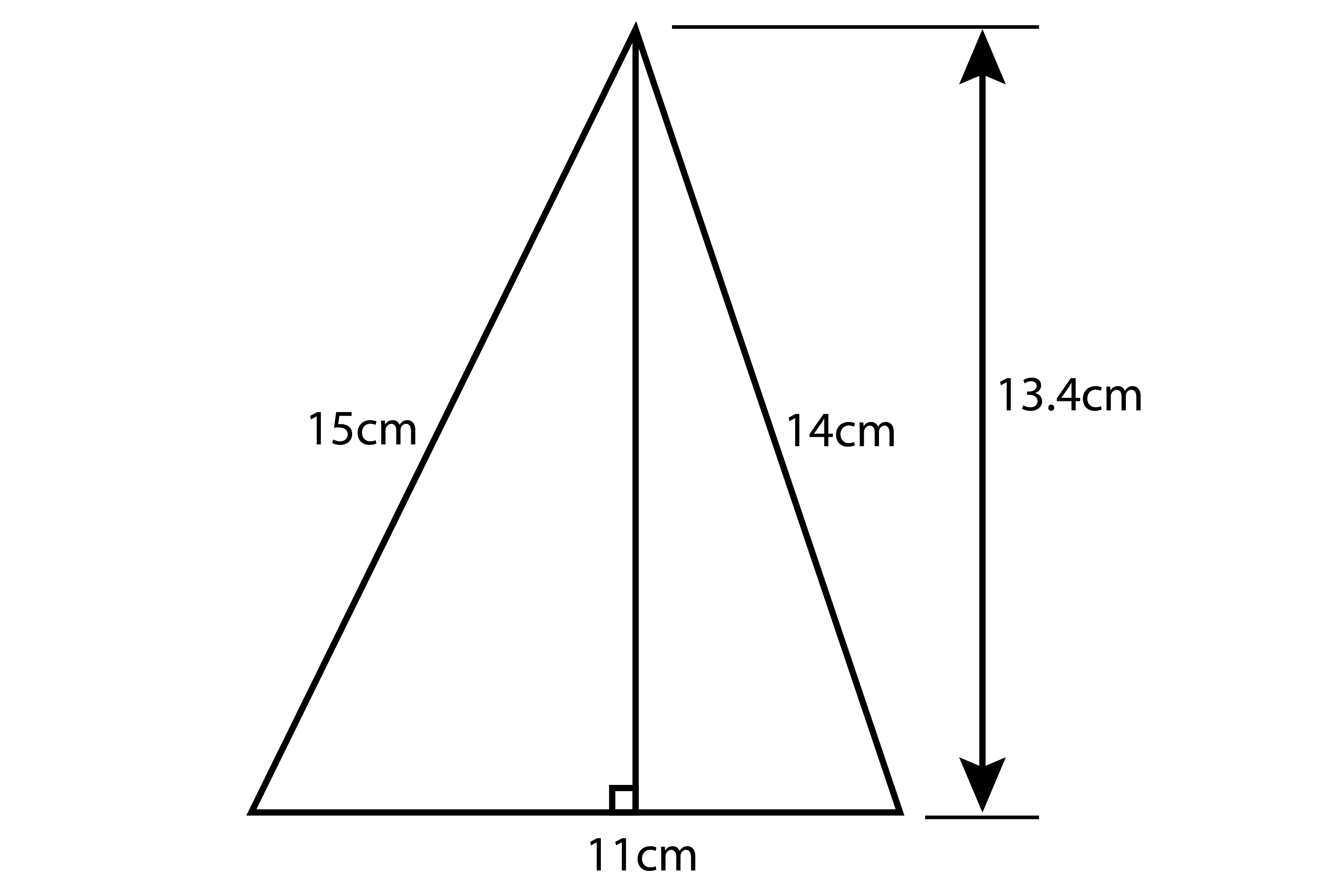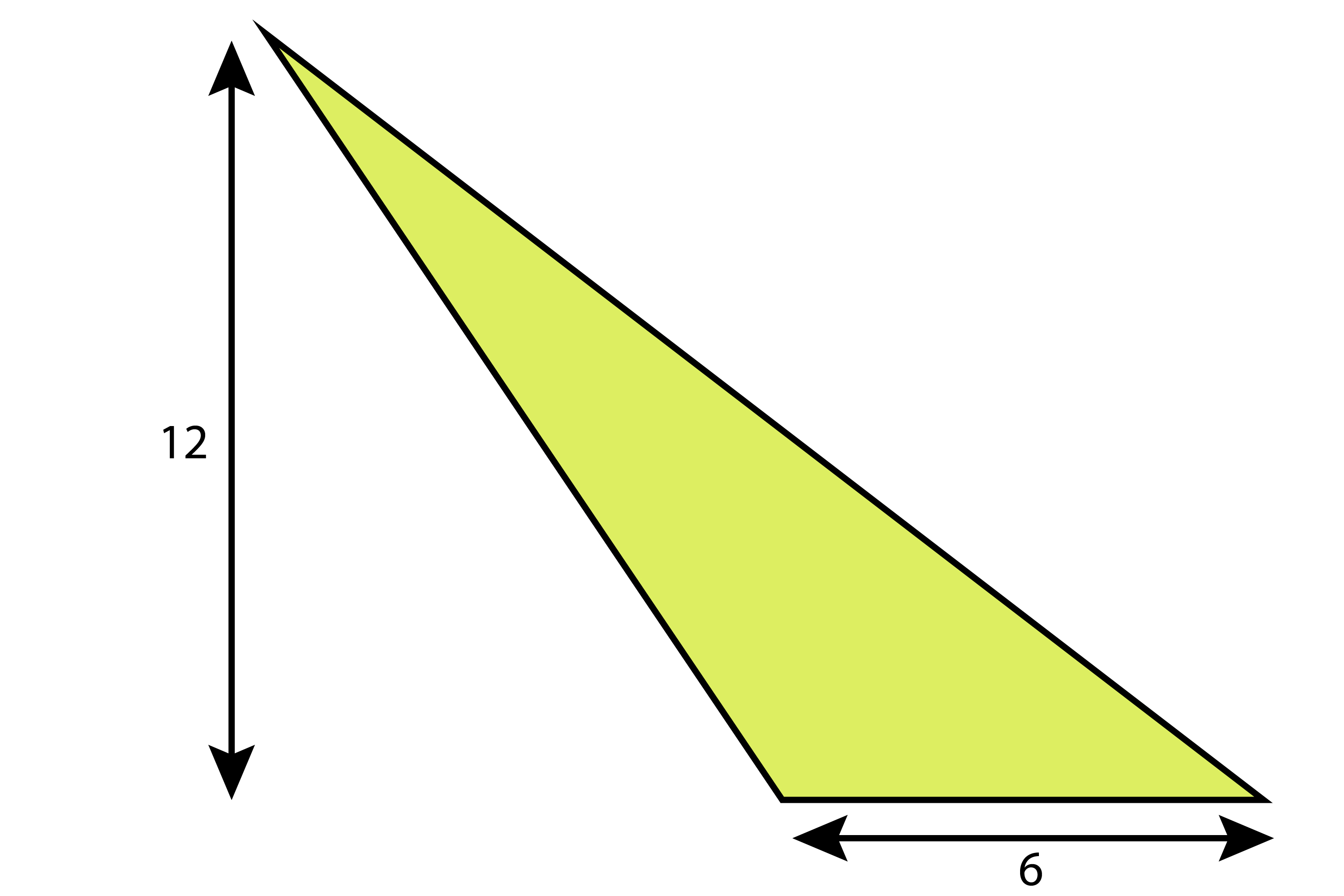# Examples of area of a triangle

Example 1

Find the area of the following triangle.Area=Basetimesheight and 1/2 it

Area=(11times13.4)/2=73.7cm^2

Example 2

What is the area of the triangle?Area=Basetimesheight and 1/2 it

Area=(6times12)/2=36